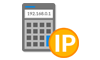##### How to determine the subnet mask if provided with the network prefix/CIDR notation:
If we are given an IP address and prefix/CIDR, the prefix/CIDR tells us how many bits of the IPv4 address is allocated to the network part of the IP address. To find out how many bits are left for the host bits, we subtract the number of bits in the CIDR notation/prefix from 32 (max number of bits in an IPv4 address).

If we are provided the network address written in CIDR as 192.168.5.0/20, we would subtract 20 from 32 (32 – 20 = 12).

To calculate the network mask if provided a prefix or the IP is written in CIDR notation, you take the prefix and write that out in binary. We would write 1s from left to right and put in zeros for all remaining bits.

If we are provided the network address written in CIDR as 192.168.5.0/20, we would write out the prefix in binary form as below, where “n” is a network bit and “h” is a host bit.

```1 1 1 1 1 1 1 1 . 1 1 1 1 1 1 1 1 . 1 1 1 1 0 0 0 0 . 0 0 0 0 0 0 0 0
n n n n n n n n . n n n n n n n n . n n n n h h h h . h h h h h h h h
```

We then convert the binary to decimal form.

The decimal number is equal to the sum of powers of 2 of the binary number ‘1’ digits place. For every bit that is flipped to 1, we will add up the sum of the powers of 2 to get the value for that octet of the subnet mask.

The subnet mask in decimal form is 255.255.240.0. For a /20 network, our subnet mask will be 255.255.240.0.